Courses

# The Collision Theory Of Bimolecular Gaseous Reaction - Chemical Kinetics Chemistry Notes | EduRev

## Chemistry : The Collision Theory Of Bimolecular Gaseous Reaction - Chemical Kinetics Chemistry Notes | EduRev

The document The Collision Theory Of Bimolecular Gaseous Reaction - Chemical Kinetics Chemistry Notes | EduRev is a part of the Chemistry Course Physical Chemistry.
All you need of Chemistry at this link: Chemistry

The Collision Theory of Bimolecular Gaseous Reaction.

The reaction between two species takes place only when they are in contact i.e. the reactant species must be collide before they react.
Consider the bimolecular elementary reaction.

A + B → P

rate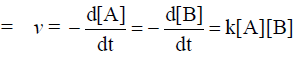The rate of reaction to be proportional to the rate of collision i.e. the mean sped of the molecules, their collision cross-section (σ) and the number of densities of A and B. Using kinetic theory of gases, the rate of bimolecular collisions per second per cm-3 between unlike molecule is given by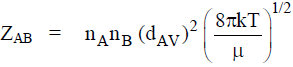Where nA & nare number of A and B molecules, dAV is the average collision diameter defined as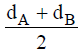and � is the reduced mass defined as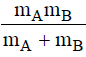ZAB = collision frequency The detailed analysis of the bimolecular collisions leads to the result that the number of collision per second per cm3 between molecules A and B is given by

rate = ZAB e-E0 / RT = no. of collision

where E0 = Energy generated by collision then the rate of relative collision is given by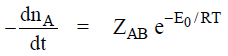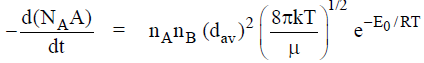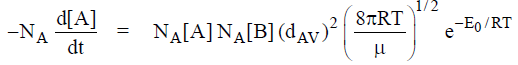∴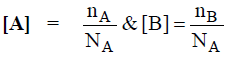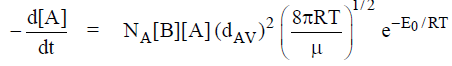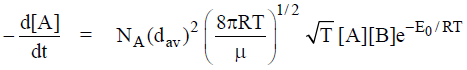let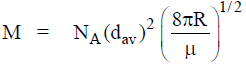then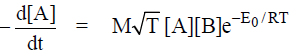…(1)

we know that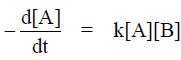then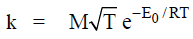…(2)

The collision theory can be generalized by introducing the steric factor, P, into the equatiohn for the bimolecular rate constant.
Then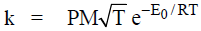Relation between Ea and E0
By between equation; k = Ae-Ea / RT
By collision theory,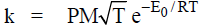Taking natural log we get ln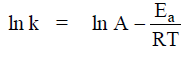…(1)

ln k = ln P + ln M + ln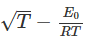…(2)

Different iate both equation (1) and (2) with respect to T we get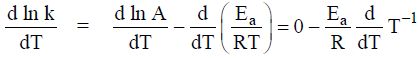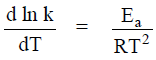.....(1a)
and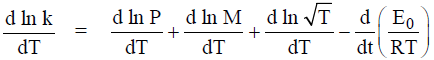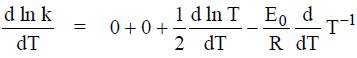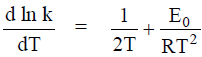…(2a)

Comparing equation (1a) & (2a) we get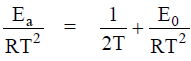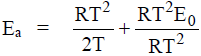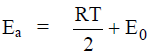…(3)

The expression for Arrhenius pre-exponential factor using collision theory We know that

k = Ae-Ea / RT                            [by Arrhenius equation]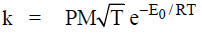[by collision theory]
then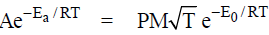…(1)
We know that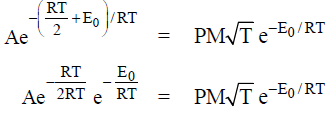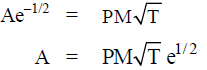…(1)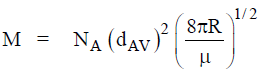then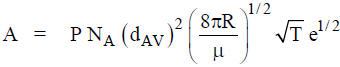…(2)

Offer running on EduRev: Apply code STAYHOME200 to get INR 200 off on our premium plan EduRev Infinity!

## Physical Chemistry

66 videos|94 docs|32 tests

,

,

,

,

,

,

,

,

,

,

,

,

,

,

,

,

,

,

,

,

,

;